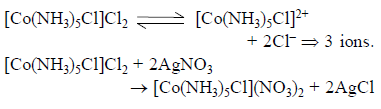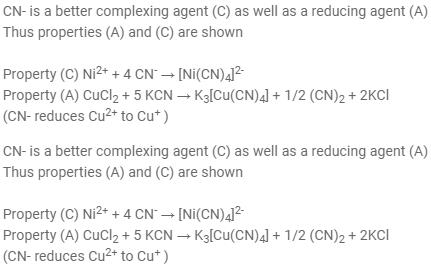Courses

# Test: Previous Year Questions - Coordination Cmpd (Level 1)

## 22 Questions MCQ Test Chemistry Class 12 | Test: Previous Year Questions - Coordination Cmpd (Level 1)

Description
This mock test of Test: Previous Year Questions - Coordination Cmpd (Level 1) for Class 12 helps you for every Class 12 entrance exam. This contains 22 Multiple Choice Questions for Class 12 Test: Previous Year Questions - Coordination Cmpd (Level 1) (mcq) to study with solutions a complete question bank. The solved questions answers in this Test: Previous Year Questions - Coordination Cmpd (Level 1) quiz give you a good mix of easy questions and tough questions. Class 12 students definitely take this Test: Previous Year Questions - Coordination Cmpd (Level 1) exercise for a better result in the exam. You can find other Test: Previous Year Questions - Coordination Cmpd (Level 1) extra questions, long questions & short questions for Class 12 on EduRev as well by searching above.
QUESTION: 1

Solution:
QUESTION: 2

Solution:
QUESTION: 3

### One mole of the complex compound Co(NH3)5Cl3, gives 3 moles of ions on dissolution in water. One mole of the same complex reacts with two moles of AgNO3solution to yield two moles of AgCl(s). The structure of the complex is –    [AIEEE-2003]

Solution:QUESTION: 4

In the coordination compound K4[Ni(CN)4], the oxidation state of nickel is –

[AIEEE-2003]

Solution:
QUESTION: 5

The number of 3d-electrons remained in Fe2+ (At. no. of Fe = 26) is –

[AIEEE-2003]

Solution:
QUESTION: 6

Ammonia forms the complex ion [Cu(NH3)4]2+ ion with copper ions in alkaline solutions but not in acidic solution. What is the reason for it –

[AIEEE-2003]

Solution:
QUESTION: 7

Among the properties (a) reducing (b) oxidising (c) complexing, the set of properties shown by CN_ ion towards metal species is –

[AIEEE-2004]

Solution:Thus correct option is A

QUESTION: 8

The coordination number of a central metal atom in a complex is determined by –

[AIEEE-2004]

Solution:
QUESTION: 9

Which one of the following complexes in an outer orbitals complex–

[AIEEE-2004]

Solution:
QUESTION: 10

Coordination compounds have great importance in biological systems. In this contect which of the following statements is incorrect ?

[AIEEE-2004]

Solution:
QUESTION: 11

The correct order of magnetic moments (spin only values in B.M. among is) –

[AIEEE-2004]

Solution:
QUESTION: 12

The value of the 'spin only' magnetic moment for one of the following configurations is 2.84 BM . The correct one is –

[AIEEE-2005]

Solution:

Spin only magnetic moment = √(n(n+2)) B.M.

Where n = number of unpaired electron

Given, √(n(n+2)) = 2.84

n(n + 2) = 8.0656

n = 2

In an octahedral complex, for a d4 configuration in a strong field ligand, number of unpaired electrons = 2

Hence A is correct.

QUESTION: 13

The IUPAC name for the complex [Co(NO2)(NH3)5] Cl2 is –

[AIEEE-2006]

Solution:
QUESTION: 14

Nickel (Z = 28) combines with a ninegative monodentate ligand X_ to form a paramagnetic complex [NiX4]2_. The number of unpaired electron in the nickel and geometry of this complex ion are respectively –

[AIEEE-2006]

Solution:
QUESTION: 15

In Fe(CO)5, the Fe–C bond possesses –

[AIEEE-2006]

Solution:
QUESTION: 16

How many EDTA (ethylenediaminetetraacetate ion) molecules are required to make an octahedral complex with a Ca2+ ion ?

[AIEEE-2006]

Solution:
QUESTION: 17

The ''spin-only'' magnetic moment [in units of Bohr magneton] of Ni2+ in aqueous solution would be (At. No. Ni = 28) –

[AIEEE-2006]

Solution:
QUESTION: 18

Which one of the following has a square planar geometry - (Co = 27, Ni = 28, Fe = 28, Fe = 26, Pt = 78) –

[AIEEE-2007]

Solution:
QUESTION: 19

The coordination number and the oxidation state of the element 'E' in the complex [E(en)2(C2O4)] NO2 (where (en) is ethylene diamine) are, respectively –

[AIEEE-2008]

Solution:
QUESTION: 20

In which of the following octahedral complexes of Co (at. no. 27), will the magnitude of Dbe the highest ?

Solution:
QUESTION: 21

Which of the following has an optical isomer ?

[AIEEE-2009]

Solution:
QUESTION: 22

Which of the following pairs represents linkage isomers ?

[AIEEE-2009]

Solution: